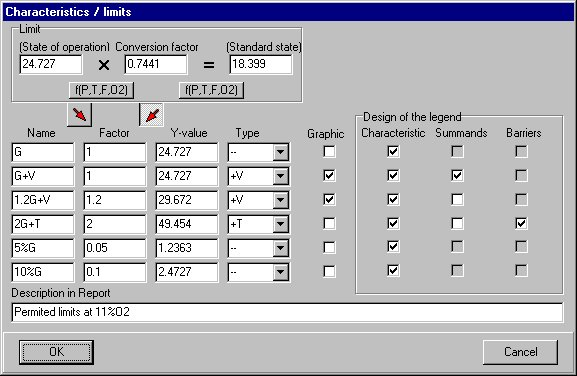# P&K 3950

## Characteristics of the measuring system

Product has been archived / Produkt wurde archiviertThe calculation refers to the Limit value (state of operation), which also can be calculated from standardization factor and Limit value (standard state), if entered. In the legend of the graphic the characteristics referring to the standard state are indicated and also possibly the factor (NormFK) which can be converted to the state of operation.

The calculation of the limit value can also be made with temperature, dampness, pressure and O2-content instead of being calculated with the factor. The appropriate form can be opened with the buttons f(P,T,F,O2). In each case, the two values above a button are calculated.

These data and some more calculated ones can be included in the report. A descriptive text can be entered in Description in Report which will then be printed below the table.

To each characteristic of the measuring system the following entries are possible:

 Name Any of 6 alphanumeric characters Factor Can be entered, in order to calculate Y-value from Factor*Limit. Y-value Y-value, which is either given or to be calculated from Factor*Limit, if these are entered. Type One type of the characteristics can be selected from a selection list: +V stands for Y-value + confidence interval and -T for Y-value -tolerance interval. Graphic When checked, the limit value will be included in the legend of the graphic. Characteristics Check box for the numerical output of the characteristic in the graphic legend. Summands Check boxes for the numerical output of the individual summands of the characteristic in the graphic legend. Barriers Check box for the output of the barrier values of the tolerance interval . Such barrier specification are shown as "x(n'<2) = lower value | upper value "in the legend.